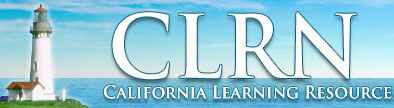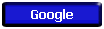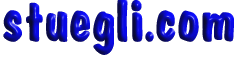# STANDARDS

## Search the Standards

### Investigation & Experimentation - Grades 9 to 12

The following content is copied from the California State Board of Education web. The main page for that site is http://www.cde.ca.gov/be/st/ss/index.asp

## Content Standards

Content standards were designed to encourage the highest achievement of every student, by defining the knowledge, concepts, and skills that students should acquire at each grade level.The content standards adopted by the California State Board of Education are listed below: Printed publications can be purchased from CDE Press Educational Resource Catalog.

• English Language Arts, Adopted December 1997
HTML | PDF (812KB; 92pp.)
HTML | PDF (814KB; 73pp.)
• History-Social Science, Adopted October 1998
HTML | PDF (848KB; 69pp.)
• Science , Adopted October 1998
HTML | PDF (539KB; 61pp.)
• Visual and Performing Arts, Adopted January 2001
Complete Document in Portable Document Format: PDF (1.7MB; 172pp.)

http://www.cde.ca.gov/be/st/ss/scmain.asp

## ScienceA Message from the State Board of Education and the State Superintendent of Public Instruction

• #### Kindergarten

Standards that all students are expected to achieve in the course of their studies are unmarked.
Standards that all students should have the opportunity to learn are marked with an asterisk (*).

## Curriculum Frameworks

Content Standards - (K-12)
Adopted by the SBE and are the basis for curriculum frameworks.

## Science Framework

Annotation, ordering information, and links to PDF files for this CDE publication.

### Science Framework for California Public Schools

The Science Framework incorporates and builds on the science content standards adopted by the State Board of Education in 1998. It is the blueprint for reform of the science curriculum, instruction, professional preparation and development, and instructional materials in California.

## Search the Content Standards Database

The following content is copied from the website noted immediately below, for the most up to date information follow the link to the source.http://www.clrn.org/home/

For the CLRN main search page - http://www.clrn.org/search

You can search the California Content Standards database by entering keywords in the search form below. Entering fewer or partial words will result in more matches.

You can also search by Standard number (example: 2.5), subject and grade. Please note that some subjects do not have standards for all grades. In this case, the standards matching the highest grade for that subject will be shown.

Please enter your search criteria below and click on the "Search Standards" button to search the database.

 Keyword(s): Standard Number: (Optional)   Example: 2.5 Subject: - (optional) - English-Language Arts History-Social Science Mathematics Science Visual & Performing Arts (Grades 9-12 only) Grade: - (optional) - K 1 2 3 4 5 6 7 8 9 10 11 12

## Grades Nine Through Twelve - Physics

Science Content Standards.

Standards that all students are expected to achieve in the course of their studies are unmarked.
Standards that all students should have the opportunity to learn are marked with an asterisk (*).

### Motion and Forces

1. Newton's laws predict the motion of most objects. As a basis for understanding this concept:
1. Students know how to solve problems that involve constant speed and average speed.
2. Students know that when forces are balanced, no acceleration occurs; thus an object continues to move at a constant speed or stays at rest (Newton's first law).
3. Students know how to apply the law F=ma to solve one-dimensional motion problems that involve constant forces (Newton's second law).
4. Students know that when one object exerts a force on a second object, the second object always exerts a force of equal magnitude and in the opposite direction (Newton's third law).
5. Students know the relationship between the universal law of gravitation and the effect of gravity on an object at the surface of Earth.
6. Students know applying a force to an object perpendicular to the direction of its motion causes the object to change direction but not speed (e.g., Earth's gravitational force causes a satellite in a circular orbit to change direction but not speed).
7. Students know circular motion requires the application of a constant force directed toward the center of the circle.
8. * Students know Newton's laws are not exact but provide very good approximations unless an object is moving close to the speed of light or is small enough that quantum effects are important.
9. * Students know how to solve two-dimensional trajectory problems.
10. * Students know how to resolve two-dimensional vectors into their components and calculate the magnitude and direction of a vector from its components.
11. * Students know how to solve two-dimensional problems involving balanced forces (statics).
12. * Students know how to solve problems in circular motion by using the formula for centripetal acceleration in the following form: a=v2/r.
13. * Students know how to solve problems involving the forces between two electric charges at a distance (Coulomb's law) or the forces between two masses at a distance (universal gravitation).

### Conservation of Energy and Momentum

1. The laws of conservation of energy and momentum provide a way to predict and describe the movement of objects. As a basis for understanding this concept:
1. Students know how to calculate kinetic energy by using the formula E=(1/2)mv2 .
2. Students know how to calculate changes in gravitational potential energy near Earth by using the formula (change in potential energy) =mgh (h is the change in the elevation).
3. Students know how to solve problems involving conservation of energy in simple systems, such as falling objects.
4. Students know how to calculate momentum as the product mv.
5. Students know momentum is a separately conserved quantity different from energy.
6. Students know an unbalanced force on an object produces a change in its momentum.
7. Students know how to solve problems involving elastic and inelastic collisions in one dimension by using the principles of conservation of momentum and energy.
8. * Students know how to solve problems involving conservation of energy in simple systems with various sources of potential energy, such as capacitors and springs.

### Heat and Thermodynamics

1. Energy cannot be created or destroyed, although in many processes energy is transferred to the environment as heat. As a basis for understanding this concept:
1. Students know heat flow and work are two forms of energy transfer between systems.
2. Students know that the work done by a heat engine that is working in a cycle is the difference between the heat flow into the engine at high temperature and the heat flow out at a lower temperature (first law of thermodynamics) and that this is an example of the law of conservation of energy.
3. Students know the internal energy of an object includes the energy of random motion of the object's atoms and molecules, often referred to as thermal energy. The greater the temperature of the object, the greater the energy of motion of the atoms and molecules that make up the object.
4. Students know that most processes tend to decrease the order of a system over time and that energy levels are eventually distributed uniformly.
5. Students know that entropy is a quantity that measures the order or disorder of a system and that this quantity is larger for a more disordered system.
6. * Students know the statement "Entropy tends to increase" is a law of statistical probability that governs all closed systems (second law of thermodynamics).
7. * Students know how to solve problems involving heat flow, work, and efficiency in a heat engine and know that all real engines lose some heat to their surroundings.

### Waves

1. Waves have characteristic properties that do not depend on the type of wave. As a basis for understanding this concept:
1. Students know waves carry energy from one place to another.
2. Students know how to identify transverse and longitudinal waves in mechanical media, such as springs and ropes, and on the earth (seismic waves).
3. Students know how to solve problems involving wavelength, frequency, and wave speed.
4. Students know sound is a longitudinal wave whose speed depends on the properties of the medium in which it propagates.
5. Students know radio waves, light, and X-rays are different wavelength bands in the spectrum of electromagnetic waves whose speed in a vacuum is approximately 3×108 m/s (186,000 miles/second).
6. Students know how to identify the characteristic properties of waves: interference (beats), diffraction, refraction, Doppler effect, and polarization.

### Electric and Magnetic Phenomena

1. Electric and magnetic phenomena are related and have many practical applications. As a basis for understanding this concept:
1. Students know how to predict the voltage or current in simple direct current (DC) electric circuits constructed from batteries, wires, resistors, and capacitors.
2. Students know how to solve problems involving Ohm's law.
3. Students know any resistive element in a DC circuit dissipates energy, which heats the resistor. Students can calculate the power (rate of energy dissipation) in any resistive circuit element by using the formula Power = IR (potential difference) × I (current) = I2R.
4. Students know the properties of transistors and the role of transistors in electric circuits.
5. Students know charged particles are sources of electric fields and are subject to the forces of the electric fields from other charges.
6. Students know magnetic materials and electric currents (moving electric charges) are sources of magnetic fields and are subject to forces arising from the magnetic fields of other sources.
7. Students know how to determine the direction of a magnetic field produced by a current flowing in a straight wire or in a coil.
8. Students know changing magnetic fields produce electric fields, thereby inducing currents in nearby conductors.
9. Students know plasmas, the fourth state of matter, contain ions or free electrons or both and conduct electricity.
10. * Students know electric and magnetic fields contain energy and act as vector force fields.
11. * Students know the force on a charged particle in an electric field is qE, where E is the electric field at the position of the particle and q is the charge of the particle.
12. * Students know how to calculate the electric field resulting from a point charge.
13. * Students know static electric fields have as their source some arrangement of electric charges.
14. * Students know the magnitude of the force on a moving particle (with charge q) in a magnetic field is qvB sin(a), where a is the angle between v and B (v and B are the magnitudes of vectors v and B, respectively), and students use the right-hand rule to find the direction of this force.
15. * Students know how to apply the concepts of electrical and gravitational potential energy to solve problems involving conservation of energy.

## Investigation & Experimentation - Grades 9 to 12

Science Content Standards.

1. Scientific progress is made by asking meaningful questions and conducting careful investigations. As a basis for understanding this concept and addressing the content in the other four strands, students should develop their own questions and perform investigations. Students will:
1. Select and use appropriate tools and technology (such as computer-linked probes, spreadsheets, and graphing calculators) to perform tests, collect data, analyze relationships, and display data.
2. Identify and communicate sources of unavoidable experimental error.
3. Identify possible reasons for inconsistent results, such as sources of error or uncontrolled conditions.
4. Formulate explanations by using logic and evidence.
5. Solve scientific problems by using quadratic equations and simple trigonometric, exponential, and logarithmic functions.
6. Distinguish between hypothesis and theory as scientific terms.
7. Recognize the usefulness and limitations of models and theories as scientific representations of reality.
8. Read and interpret topographic and geologic maps.
9. Analyze the locations, sequences, or time intervals that are characteristic of natural phenomena (e.g., relative ages of rocks, locations of planets over time, and succession of species in an ecosystem).
10. Recognize the issues of statistical variability and the need for controlled tests.
11. Recognize the cumulative nature of scientific evidence.
12. Analyze situations and solve problems that require combining and applying concepts from more than one area of science.
13. Investigate a science-based societal issue by researching the literature, analyzing data, and communicating the findings. Examples of issues include irradiation of food, cloning of animals by somatic cell nuclear transfer, choice of energy sources, and land and water use decisions in California.
14. Know that when an observation does not agree with an accepted scientific theory, the observation is sometimes mistaken or fraudulent (e.g., the Piltdown Man fossil or unidentified flying objects) and that the theory is sometimes wrong (e.g., the Ptolemaic model of the movement of the Sun, Moon, and planets).Copyright © 2005 -  S. B. EglI